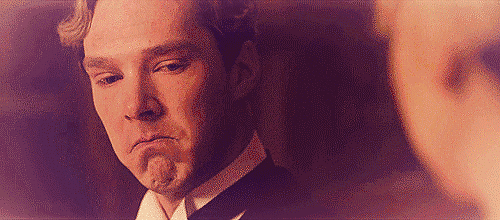## 重磅！生二胎国家奖励一套房产？！@郑州人，你敢不敢生？

2018/08/09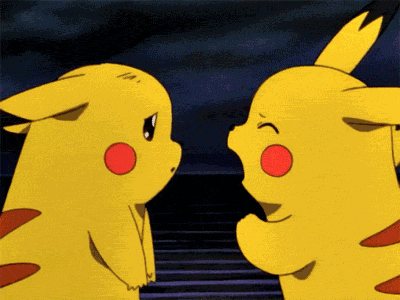（宝宝心里苦啊~）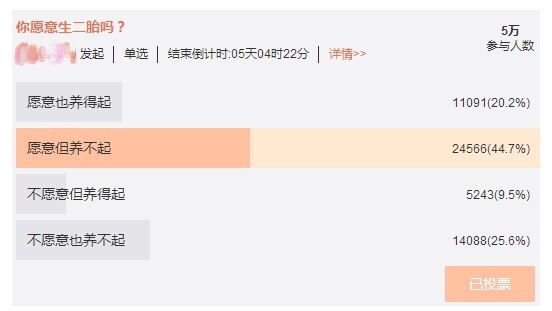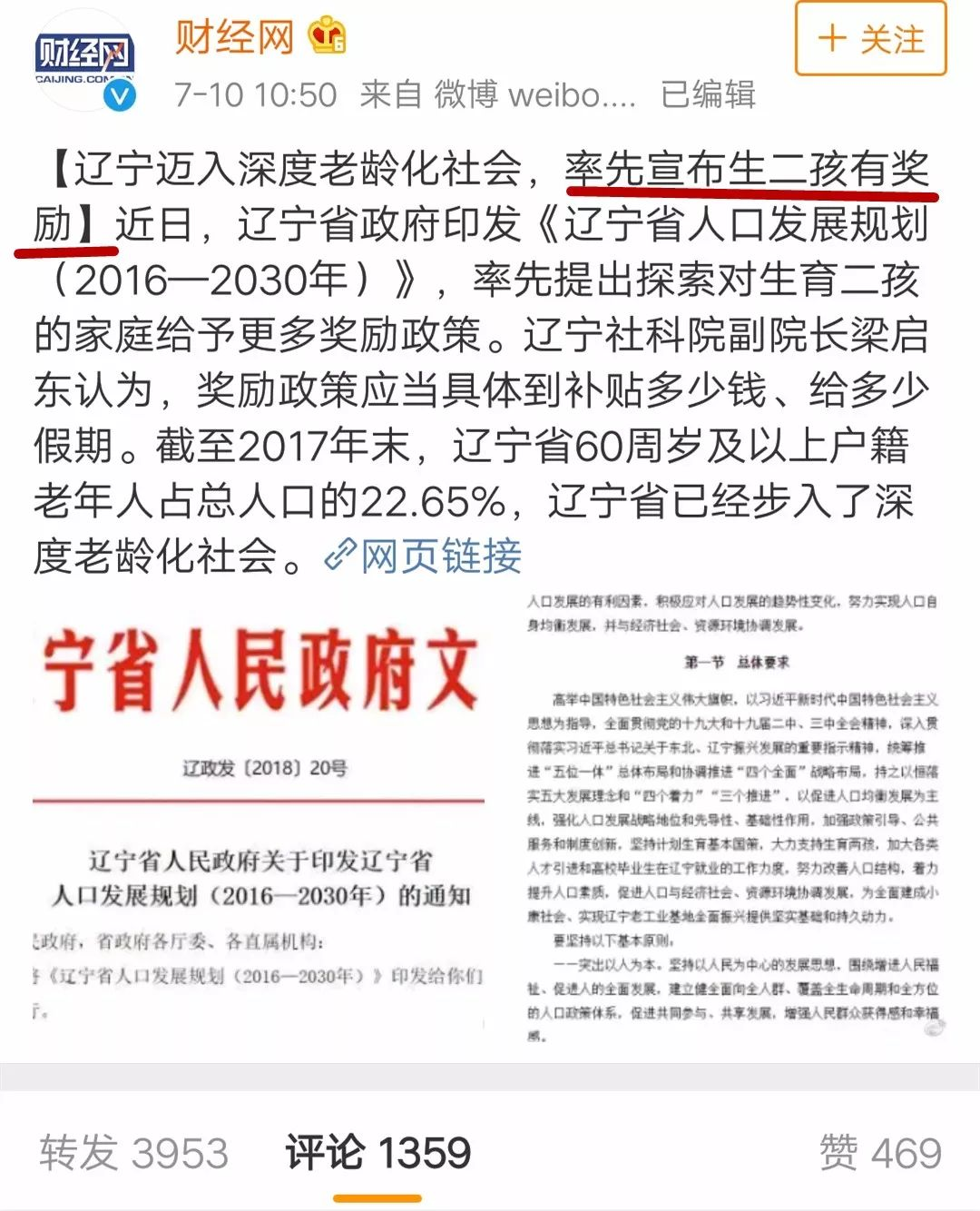▼▼▼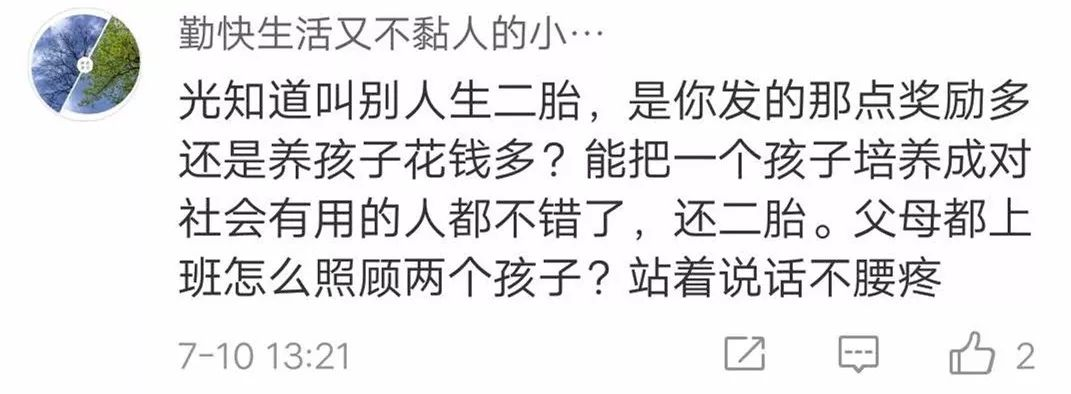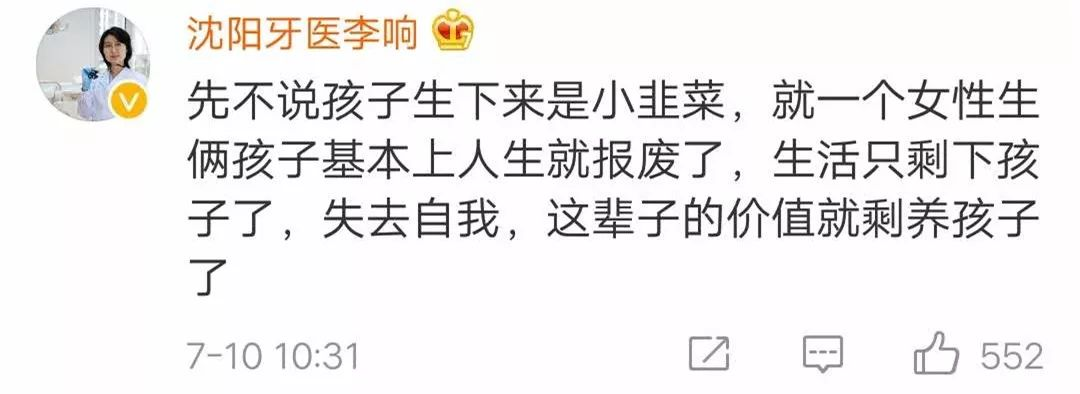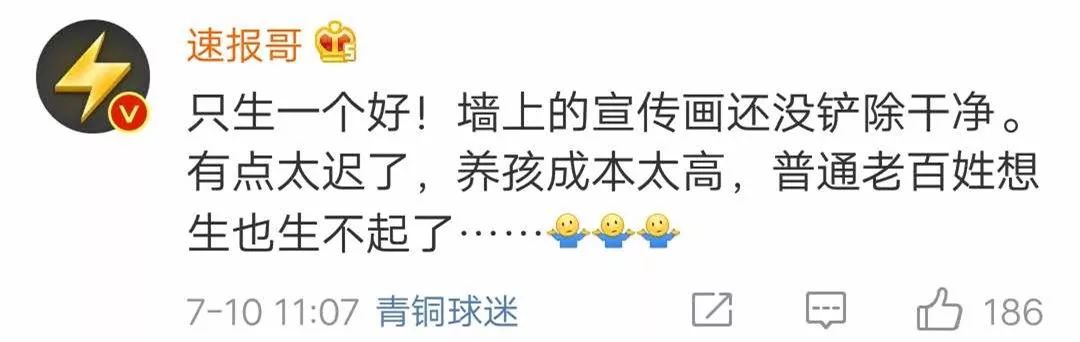▼▼▼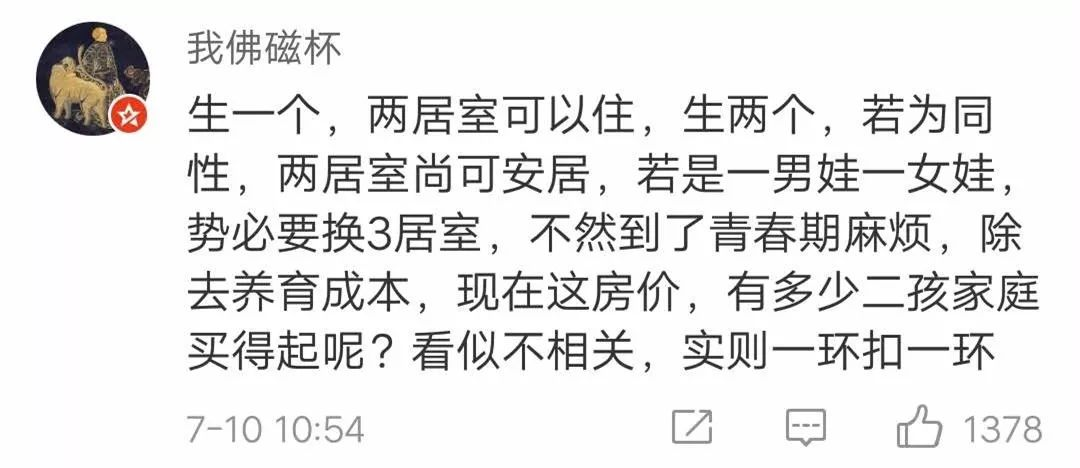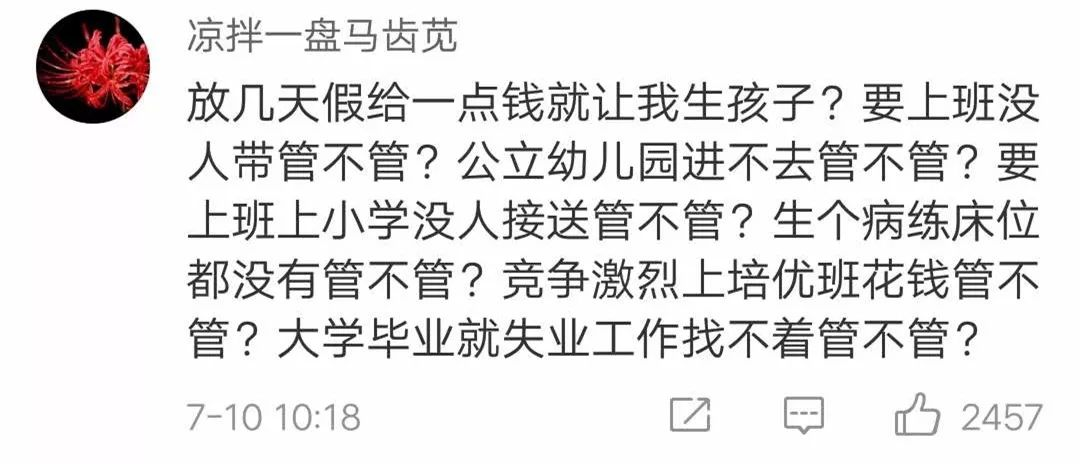▼▼▼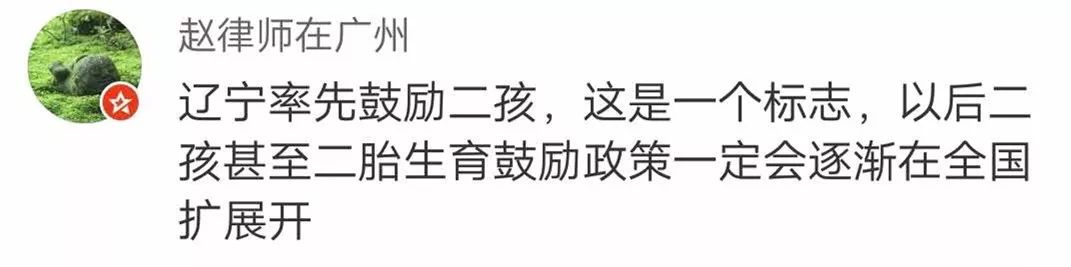（而喵姐就不一样了，优秀贵族~）▼▼▼

6000+4000+3000+6000=19000元

0-3岁 费用

▼▼▼

0-1岁

3000×12个月=36000

2000×12个月=24000元

1-3岁

5000+1000×24个月=29000元

36000+29000=65000元

▼▼▼

2000×12×3=72000元

（注意，此处是按照3年来算，

▼▼▼

（还是一周一次课的）

2000×6年+3000×6年+5000×6年=90000元

▼▼▼

(4000+20000)×6学期=144000元

12000+5000）×6学期=102000元

144000+15000+30000=189000元

▼▼

1000+1000+1000+）×6学期

+18000×3+20000×6=192000元

▼▼

20000+2000×48个月+2000×4=124000元

▼▼▼

74×2=148万！

74×2=148万！

74×2=148万！